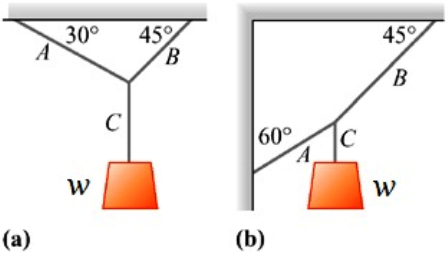# Find the tension in each cord in the figure if the weight of the suspended object is W=390N

Find the tension in each cord in the figure if the weight of the suspended object is W=390N
1.) Find the tension in cord A for system (a) and (b)
2.) Find the tension in cord C for system (a) and (b).
3.) Find the tension in cord B for system (a) and (b).